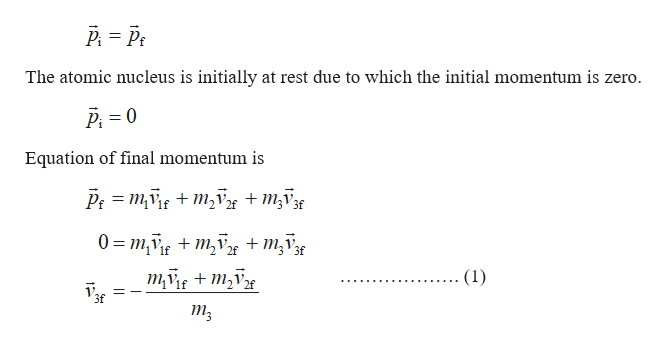# An unstable nucleus of mass 1.7 x 10-26 kg, initially atrest at the origin of a coordinate system, disintegrates intothree particles. One particle, having a mass of m1 = 5.0 x 10-27 kg, moves in the positive y- direction with speed v 1 =6.0x 106 m/s. Another particle, of mass m2 = 8.4 x 10-27 kg, moves in the positive x - direction with speed v2 = 4.0 x 106 m/s. Find the magnitude and direction of the velocity of thethird particle.

Question
1 views

An unstable nucleus of mass 1.7 x 10-26 kg, initially at
rest at the origin of a coordinate system, disintegrates into
three particles. One particle, having a mass of m1 = 5.0 x 10-27 kg, moves in the positive y- direction with speed v 1 =6.0x 106 m/s. Another particle, of mass m2 = 8.4 x 10-27 kg, moves in the positive x - direction with speed v2 = 4.0 x 106 m/s. Find the magnitude and direction of the velocity of the
third particle.

check_circle

Step 1

Write the equation of conse...help_outlineImage TranscriptioncloseP. = P: The atomic nucleus is initially at rest due to which the initial momentum is zero. P = 0 Equation of final momentum is P: = mf + m,V + M;V3g 0 = m,v + m,vx +m;V3g mg + m,V (1) 1f 3f fullscreen

### Want to see the full answer?

See Solution

#### Want to see this answer and more?

Solutions are written by subject experts who are available 24/7. Questions are typically answered within 1 hour.*

See Solution
*Response times may vary by subject and question.
Tagged in
SciencePhysics

### Work,Power and Energy